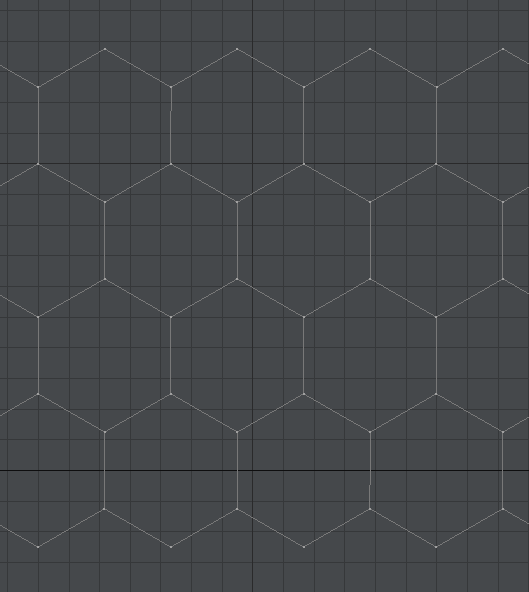What is the best tools/method to draw honeycombs(hexagon)?

Recommended Posts

What is the best tools/method to draw honeycombs(hexagon)?

and 3D hexagons, animation hexagons.

Share on other sites

1 hour ago, PeterBushMan said:

What is the best tools/method to draw honeycombs(hexagon)?

Where?

In a 3D app?

In OpenGL/Direct3D?

In the Unity/Unreal etc. game engine?

In C/C++/C#/Java etc. ?

C/C++ code:

void moveTo( int x, int y ) {
// fill me
}
void lineTo( int x, int y ) {
// fill me
}
void hexagon( int x, int y, int radius ) {
for( int i = 0; i < 6; i++ ) {
double angle1 = 2.0 * M_PI * 360.0 * i / 6;
double angle2 = 2.0 * M_PI * 360.0 * ( i + 1 ) / 6;
moveTo( x + sin( angle1 ) * radius, y + cos( angle1 ) * radius );
lineTo( x + sin( angle2 ) * radius, y + cos( angle2 ) * radius );
}
}

In the 3D application. I used the Sphere tool, set Z to 0 to have a circle, changed the number of segments to 6, and then used the Array tool:Edited by Sensei

Create an account

Register a new account